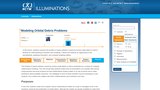Updating search results...

# 2 Results

View
Selected filters:
• NCTMRestricted Use
Rating
0.0 stars

Students use data on space debris, analysis of rates,  and regression in order to model situations.

Subject:
Mathematics
Material Type:
Alternate Assessment
Interim/Summative Assessment
Lesson Plan
Provider:
NCTM
04/18/2016Restricted Use
Rating
0.0 stars

"The Knots Lab" is a hands-on experiment that can be used when teaching linear functions; primarily when discussing lines of best fit.  Students are given a rope (3-5 feet in length) and a ruler.  Students begin by measuring the rope.  Students then tie knots in the rope, and after tying each knot, measure the resulting length of the rope.  After constructing a scatter plot of their data, students are asked a series of questions where they must use their data and regression equation to answer.

Subject:
Mathematics
Material Type: